Constructing Triangle - Given base, angle and sum of other 2 sides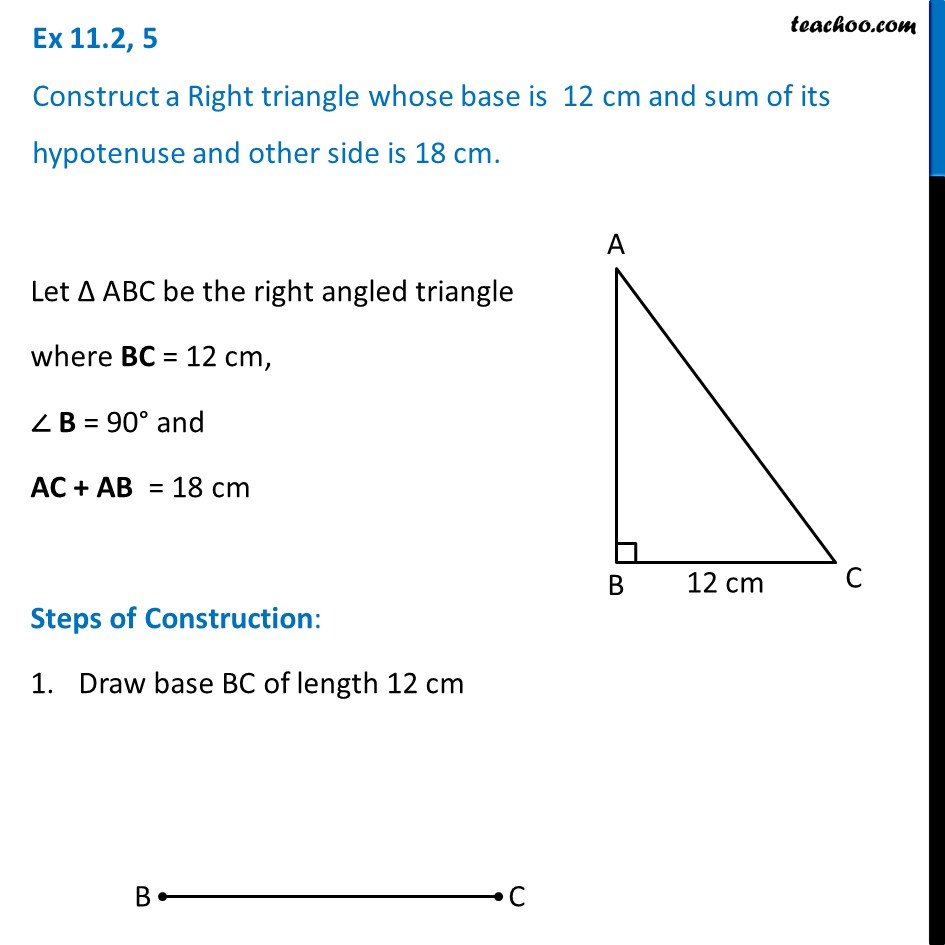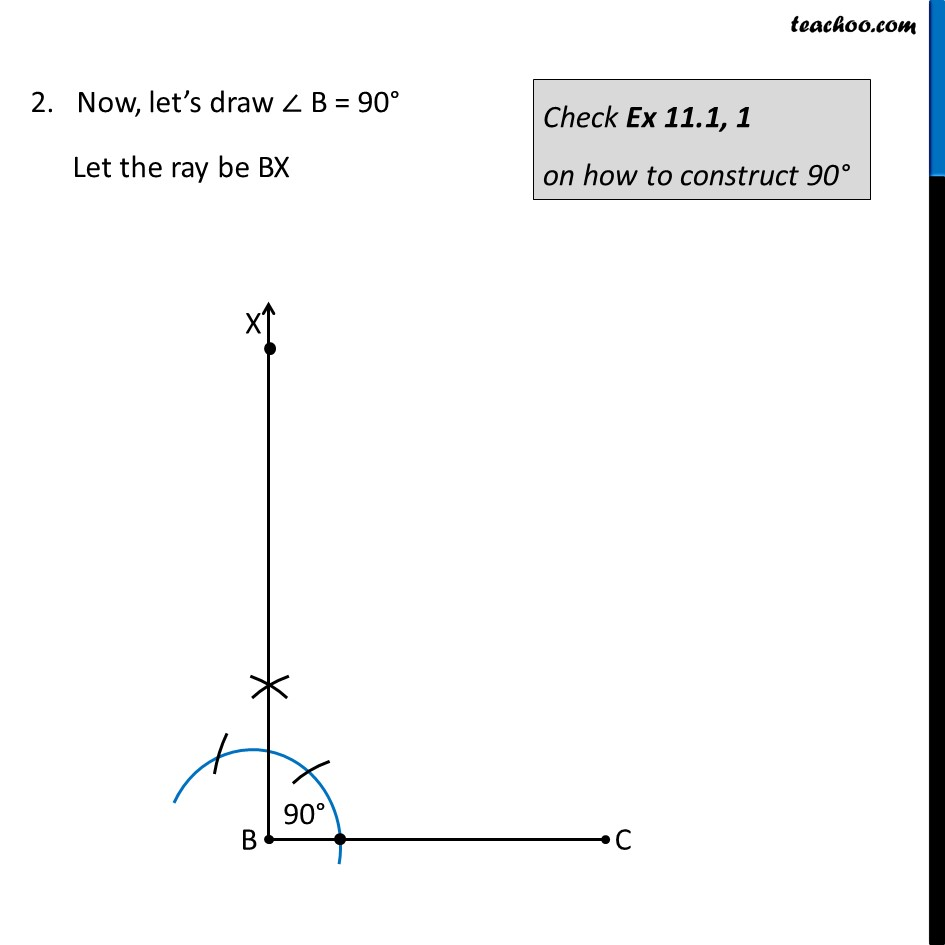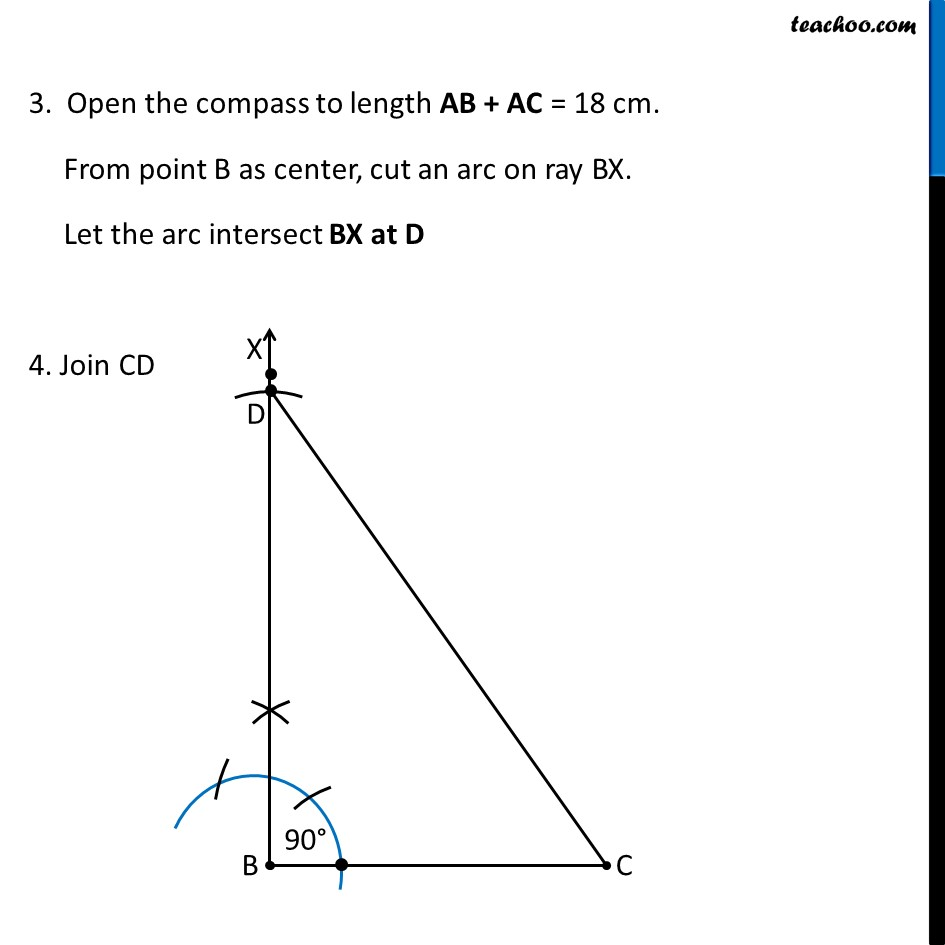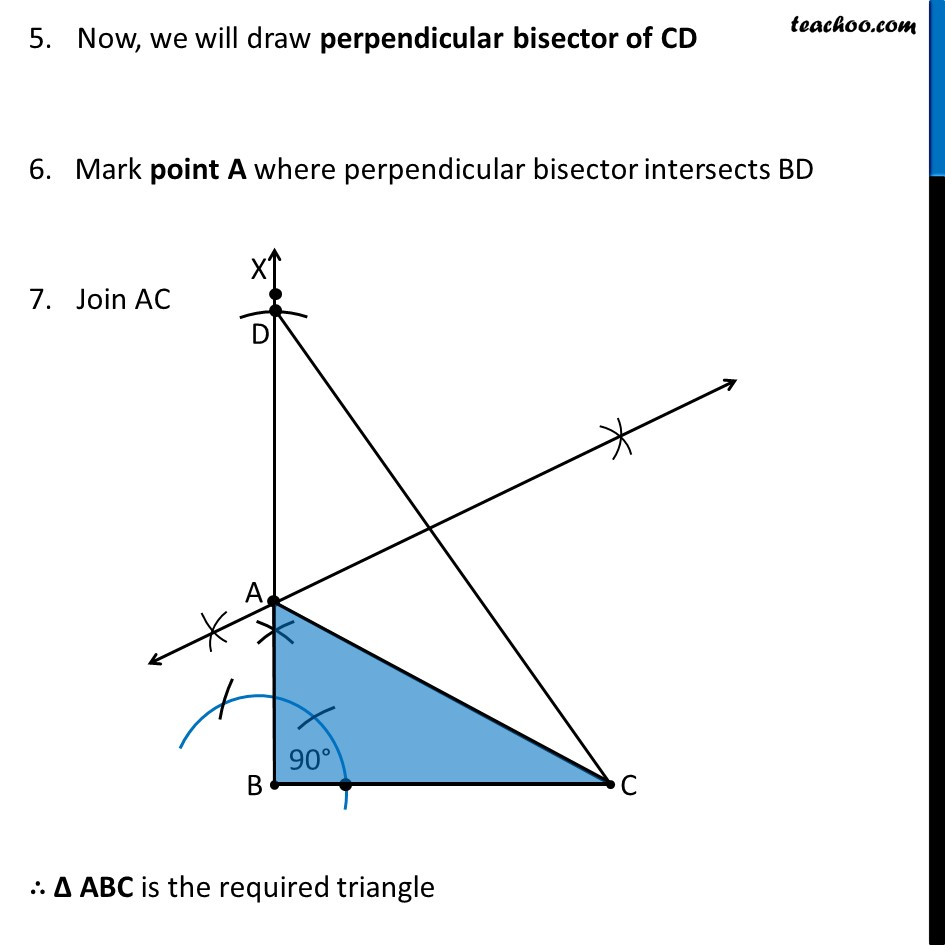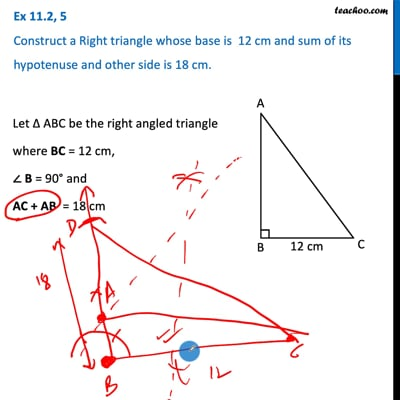This video is only available for Teachoo black users

Introducing your new favourite teacher - Teachoo Black, at only ₹83 per month

### Transcript

Ex 11.2, 5 Construct a Right triangle whose base is 12 cm and sum of its hypotenuse and other side is 18 cm. Let Δ ABC be the right angled triangle where BC = 12 cm, ∠ B = 90° and AC + AB = 18 cm Steps of Construction: Draw base BC of length 12 cm 2. Now, let’s draw ∠ B = 90° Let the ray be BX Check Ex 11.1, 1 on how to construct 90° 3. Open the compass to length AB + AC = 18 cm. From point B as center, cut an arc on ray BX. Let the arc intersect BX at D 4. Join CD Now, we will draw perpendicular bisector of CD 6. Mark point A where perpendicular bisector intersects BD Join AC ∴ Δ ABC is the required triangle